Updating search results...

# 10 Results

View
Selected filters:Rating
0.0 stars

Students compare the relationships between radius, diameter, circumference, and area by manipulating a circle and increasing or decreasing its size in this interactive from Illuminations. It also provides a problems section where students determine the answer to each question using the applet, a calculator, or paper and pencil. They must also indicate the proper units!

Subject:
Geometry
Mathematics
Material Type:
Activity/Lab
Interactive
Provider:
National Council of Teachers of Mathematics
Author:
Illuminations
02/26/2019Rating
0.0 stars

The Greek mathematician Archimedes approximated pi by inscribing and circumscribing polygons about a circle and calculating their perimeters. Similarly, the value of pi can be approximated by calculating the areas of inscribed and circumscribed polygons. This activity allows for the investigation and comparison of both methods.

Subject:
Mathematics
Material Type:
Activity/Lab
Interactive
Provider:
National Council of Teachers of Mathematics
Author:
Illuminations
02/26/2019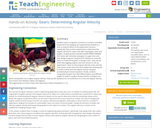Educational Use
Rating
0.0 stars

Students work as engineers and learn to conduct controlled experiments by changing one experimental variable at a time to study its effect on the experiment outcome. Specifically, they conduct experiments to determine the angular velocity for a gear train with varying gear ratios and lengths. Student groups assemble LEGO MINDSTORMS(TM) NXT robots with variously sized gears in a gear train and then design programs using the NXT software to cause the motor to rotate all the gears in the gear train. They use the LEGO data logging program and light sensors to set up experiments. They run the program with the motor and the light sensor at the same time and analyze the resulting plot in order to determine the angular velocity using the provided physics-based equations. Finally, students manipulate the gear train with different gears and different lengths in order to analyze all these factors and figure out which manipulation has a higher angular velocity. They use the equations for circumference of a circle and angular velocity; and convert units between radians and degrees.

Subject:
Applied Science
Engineering
Material Type:
Activity/Lab
Provider:
TeachEngineering
Provider Set:
TeachEngineering
Author:
James Cox
Jasmin Mejias
Jennifer S. Haghpanah
Leonarda Huertas
Mihai Pruna
09/18/2014Rating
0.0 stars

This classroom activity, which centers on documenting the location of a meteorite in Antarctica, introduces students to the technology of Global Positioning Systems. The printable handout includes a series of inquiry-based questions to get students thinking about the challenges of marking exact positions in an area where the landscape is continually changing, illustrated activity directions and a worksheet that guides students through the way satellites are used in GPS and includes areas for recording their findings.

Subject:
Science
Material Type:
Activity/Lab
Diagram/Illustration
Lesson Plan
Simulation
Provider:
American Museum of Natural History
Author:
American Museum of Natural History
Rice University
07/31/2019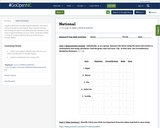Conditional Remix & Share Permitted
CC BY-NC
Rating
0.0 stars

Students will practice measuring the diameter and radius of food themed circular objects. Next, they will discover that finding the ratio of circumference and diameter is the value of approximately 3.14 or pi.
Then, using the number 3.14 for Pi, students will find the area of the various circular objects.

Subject:
Algebra
Geometry
Math 1
Mathematics
Material Type:
Activity/Lab
Homework/Assignment
07/10/2020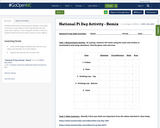Conditional Remix & Share Permitted
CC BY-NC
Rating
0.0 stars

Students will practice measuring the diameter and radius of various circular objects. Next, they will discover the ratio of pi to about 3.
Then, using the number 3.14 for Pi, students will find the area of the various circular objects.

Subject:
Algebra
Geometry
Math 1
Mathematics
Material Type:
Activity/Lab
Homework/Assignment
11/06/2019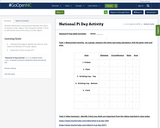Conditional Remix & Share Permitted
CC BY-NC
Rating
0.0 stars

Students will practice measuring the diameter and radius of various circular objects. Then using the number 3.14 for Pi, will find the area of the various circular objects.

Subject:
Algebra
Geometry
Math 1
Mathematics
Material Type:
Activity/Lab
Homework/Assignment
11/06/2019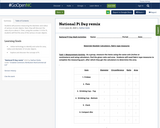Conditional Remix & Share Permitted
CC BY-NC
Rating
0.0 stars

Students will practice measuring the diameter and radius of various circular objects. Next, they will discover the ratio of pi to about 3.
Then, using the number 3.14 for Pi, students will find the area of the various circular objects.

Subject:
Algebra
Geometry
Math 1
Mathematics
Material Type:
Activity/Lab
Homework/Assignment
07/07/2020Rating
0.0 stars

Lesson plan where students are asked to consider how to draw a perfect circle, and learn to do so using a line segment called a radius. They also learn to fold a circle in two directions symmetrically to find its center. This activity is motivated by a CYBERCHASE episode in which the CyberSquad set out to rescue a magic ring from Hacker and deposit it in a safe place.

Subject:
Geometry
Mathematics
Material Type:
Activity/Lab
Lesson Plan
Presentation
Provider:
WGBH - Teachers' Domain
Author:
WNET.org
02/26/2019Rating
0.0 stars

In this video segment from Cyberchase, the CyberSquad is trapped in one of the Spheres of Fears, and they are trying to get through a gate to escape. The key to the gate is locked inside a can, and in order to access the key they must attach the correct label to the can. After guessing incorrectly on the first try, the CyberSquad decides to take some measurements to find the correct label. Using two circles nearby, they compare the diameter of each circle to its circumference. They realize there is a special relationship between the circumference of a circle and the length of its diameter. Teaching tips are also provided that discuss frame, focus and follow-up suggestions for using this video in a math lesson.

Subject:
Geometry
Mathematics
Material Type:
Activity/Lab
Presentation
Provider:
WGBH - Teachers' Domain
Author:
WNET.org